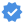#### Physic

n/a

If You Find Any Mistakes On Our Content or Want To make some Changes Then Report Now

# Physics

## Physic Class 11 NEB Syllabus [ UPDATED ]

### Physical Quantities

1. Demonstrate the meaning, importance and applications of precision in the measurements.
2. Understand the meaning and importance of significant figures in measurements.
3.  Explain the meaning of dimensions of a physical quantity.
4. Workout the dimensions of derived physical quantities applicable to this syllabus.
5. Apply dimensional analysis method to check the homogeneity of physical equations.

### Vectors

1.  Distinguish between scalar and vector quantities.
2. Add or subtract coplanar vectors by drawing scale diagram (vectortriangle,parallelogram or polygon method).
3. Understand the meaning and importance of unit vectors.
4. Represent a vector as two perpendicular components.
5. Resolve co-planer vectors using component method.

Kinematics

1. Define displacement, instantaneous velocity and acceleration with relevant examples.
2. Explain and use the concept of relative velocity.
3. Draw displacement-time and velocity-time graph to represent motion, and determine velocity from the gradient of displacement-time graph, acceleration from the gradient of velocity-time graph and displacement from the area under a velocity-time graph.
4. Establish equations for a uniformly accelerated motion in a straight line from graphical representation of such motion and use them to solve related numerical problems.
5. Write the equations of motion under the action of gravity and solve numerical problem related to it.
6. Understand projectile motion as motion due to a uniform velocity in one direction and a uniform acceleration in a perpendicular direction, derive the equations for various physical quantities (maximum height, time of flight, time taken to reach maximum height, horizontal range, resultant velocity) and use them to solve mathematical problems related to projectile motion.

## Dynamics

1. Define linear momentum, impulse, and establish the relation between them.
2. Define and use force as rate of change of momentum.
3. State and prove the principle of conservation of linear momentum using Newton’s second and Newton’s third of motion.
4. Define and apply moment of a force and torque of a couple.
5. State and apply the principle of moments
6. State and apply the conditions necessary for a particle to be in equilibrium.
7. State and explain the laws of solid friction.
8. Show the coefficient of friction is equal to the tangent of angle of repose and use the concept to solve problems.
9. Solve the numerical problem and conceptual question on dynamics

## Work, energy and power

1. Explain work done by a constant force and a variable force.
2. State and prove work-energy theorem.
3. Distinguish between kinetic energy and potential energy and establish their formulae.
4. State and prove the principle of conservation of energy.
5. Differentiate between conservative and non-conservative force.
padandasModefied ByGandaki College of Engineering and Science (GCES)Pokhara,Lamachaur
+9846790567Gandaki College of Engineering and Science (GCES)Pokhara,Lamachaur
+9846790567Gandaki College of Engineering and Science (GCES)Pokhara,Lamachaur
+9846790567

## Contents

We just added New Courses Notes Pdf

Search Pdf